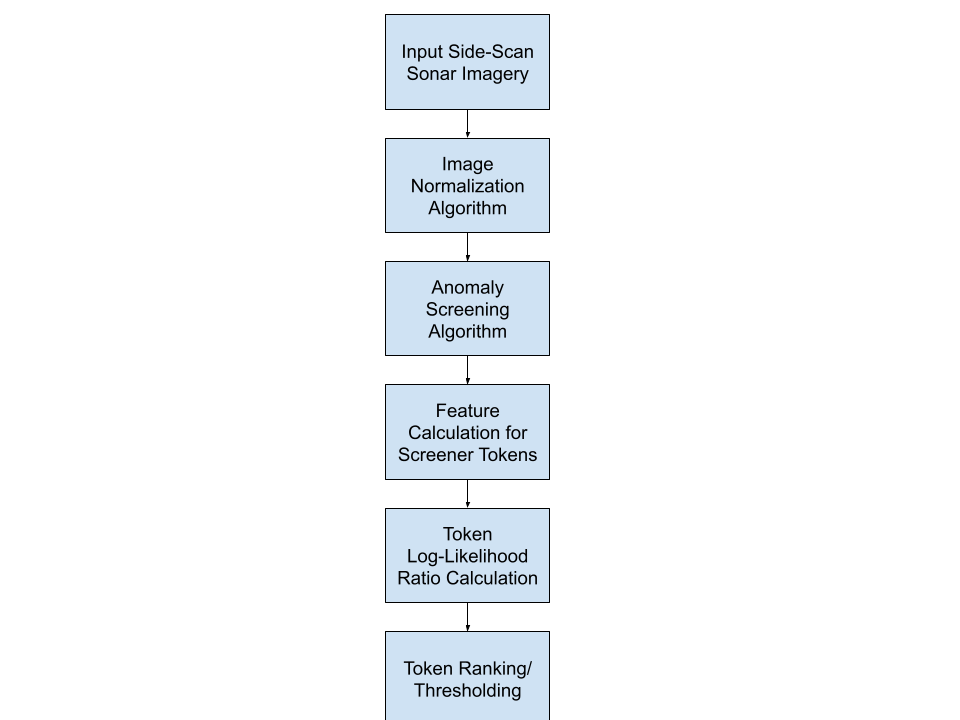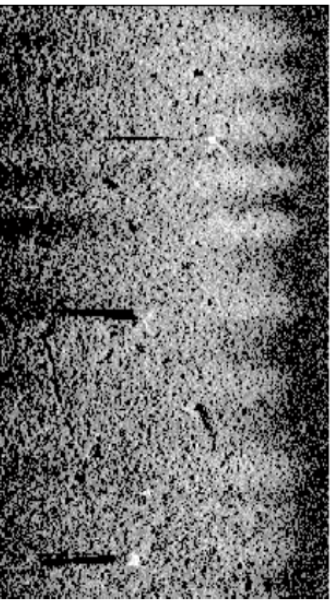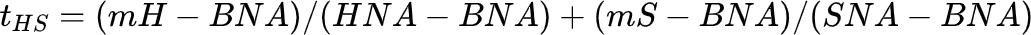# Hunting Submarine Mines

### Hunting Submarine Mines: A Comparison of Support Vector Machines and Multilayer Perceptron Networks in Building Mine Classification Models

A summary of the correspondent Hunting Submarine Mines paper published by Martin G. Bello and Gerald J. Dobeck

### General Information

The presented paper on hunting submarine mines is about automated mine detection, comparing the original approach of applying a Multilayer Perceptron Network with an alternative method of applying a Support Vector Machine to conduct the classification process of hunting submarine mines. Moreover, necessary steps before the classification can be executed such as image normalization, anomaly screening, and feature calculation are described respectively. Eventually, the performance of each method is compared and evaluated.Figure 1 Aggregate Detection/Classification Algorithm Structure (Bello & Dobeck, 2003, pp.2)

### Image processing with normalization and anomaly screening

For the first step, obtaining side-scan sonar imagery, the Bluefin BPAUV/ Klein Sonar was used. It creates input images that are then processed in the algorithm described in figure 1. The comparison of unprocessed images and ones where normalization and anomaly screening was applied can be seen in figure two with the unprocessed one on the left and the processed one on the right.

Figure 2 comparison of an image before and after augmentationIt can be observed that the processed one has Mine-Like objects highlighted and the contrast between background and object intensified. The purpose of the image normalization part is to estimate local background intensity at each pixel. This results in highlights and shadows being more visible. In this very example, the normalization algorithm developed by Dr. Gerald Dobeck of the Naval Surface Warfare Center Coastal System Station was used. In the following step, the anomaly screening, the goal is to extract “blobs”. In this paper, blobs are referred to as collections of connected pixels, which correspond to Mine-Like objects. These candidate Mine-Like blobs are then referred to as image Tokens

### The anomaly screening algorithm.

As already mentioned, anomaly screening is used to extract connected pixels that can be identified as Mine-Like objects. An analysis of the used image database showed that Mine-Like targets have relatively small highlight regions, followed by larger shadows. Thus, a highlight/shadow contrast is built and used as an anomaly statistic. The following equation shows how the contrast is being determined:with mHand mS representing the intensity of the Highlight and the Shadow in a certain window, BNA, HNA and SNA representing the average intensity of Background, Highlight, and Shadow.

After the corresponding statistics have been computed, the anomaly statistic cumulative distribution function, Fcda, is determined, and pixels are extracted. Here, the following condition has to be true:

with ta being an anomaly statistic and denoting the top percentage of pixels to be extracted.The final tokens that are then returned by the anomaly screening algorithm are those for which the following conditions are true:

And

with the Thresh_screener just being a threshold the aggregated rank is not supposed to surpass.

The anomaly-screening algorithm explained above is actually a general version that was even further extended in the paper. In order to maximize the accuracy of the detector, three different anomaly statistics are computed. In addition to the t_HS statistic, that has already been explained, t_H and t_S, which are explicit Highlight and Shadow anomaly statistics, are determined as shown below:whereas the indices i and j denote the center of a local rectangular, n(i,j) denotes an average of squared deviations within its neighborhood, which is according to a previous version of the paper two pixels in each direction, and nM is a median value for n(i,j). Lastly, after t_HS and t_H have been computed, shadow statistic t_S is calculated as follows:

where I_N(i,j) denotes the average intensity of the normalized image.

(Bello & Dobeck, 2003, pp.3-5)### The feature selection as well as augmentation

In the paper, the feature vector fBis 23-dimensional and uses t_HS, t_H, t_S, and the normalized image I_N in order to compute the 23 features. All of the features are listed below:

f1 = PC for HS-segmentation

f2 = min(rPC,rMP) for HS-segmentation

f3 = “local” summation of unfiltered blob PC for HS-segmentation

f4 = mean t_HS over blob for HS-segmentation

f5 = standard deviation of unfiltered blob count for HS-segmentation

f6 = “local” summation of unfiltered blob count for HS-segmentation

f7 = MP for t_HS over blob for HS-segmentation

f8 = (1,0) valued indicator for existence of intersecting H-segmentation blob

f9 = MP for t_H over intersecting H-segmentation blob

f10 = PC for intersecting H-segmentation blob

f11 = (1,0) valued indicator for existence of intersecting S-segmentation blob

f12 = PC for intersecting S-segmentation blob

f13 = mean I_N over blob for HS-segmentation

f14 = maximum I_N over blob for HS-segmentation

f15 = standard deviation of I_N over blob for HS-segmentation

f16 = skewness coefficient of I_N over blob for HS-segmentation

f17 = kurtosis coefficient of I_N over blob for HS-segmentation

f18 = perimeter of blob for HS-segmentation

f19 = (16*PC)/(perimeter*perimeter) for HS-segmentation blob

f20 = perimeter/(2*(bounding-box-width + bounding-box-height)) for HS-segmentation blob

f21 = (major-axis – minor-axis) / (major-axis + minor-axis) for HS-segmentation blob

f22 = major-axis

f23 = orientation of HS-segmentation blob

Features f1-f7 are adopted from the original feature set from 1992, features f8-f10 are Highlight related, features f11-f12 are Shadow related, features f13-f17 are statistical intensity distribution related, and features f18-f23 are shape related.

As a next step, those features are augmented with Two-Dimensional Discrete Cosine Transform and Pseudo-Zernike Moment Feature Definition. (Bello & Dobeck, 2003, pp.5-8)

### Comparison of the MLP and SVM classifier construction

Both algorithms are trained with a feature vector X and a result vector Y, whereas Y consists of two classes, +1 for class 1 and -1 for class 2. The algorithm tries to optimize mutual information between a feature vector and a “True” class by first going through a certain amount of hidden layers where activations are computed followed by a backward pass where the parameter vector 𝛀 is adapted based on the calculated error.

Furthermore, a test set is used for cross-validation which helps to terminate the training when the integral over a Test Set Derived Receiver Operating Characteristic Curve (ROC) is maximized. Moreover, an epoch size of 50-100 appears to result in the best outcome.

For the Support Vector Machine approach, the SVM Light implementation by Professor Thorsten Joachims was used. Here, a linear activation, as well as radial basis function, were applied. Furthermore, slack-variables denoted as 𝝺 are defined in order to allow misclassification. Moreover, a regularization parameter is defined as C which helps to control the bias/variance ratio. (Bello & Dobeck, 2003, pp.8-11)

### Feature selection and comparison of MLP and SVM performance results for Hunting Submarine Mines

Due to the fact that feature augmentation created an extremely large feature set, a Genetic Algorithm (GA), to be more precise, a NeuralWare predict algorithm, is used in order to determine a set of features that achieves the best results. The conclusion that will follow shortly is based on comparing the MLP and the SVM algorithm as well as the linear SVM and the non-linear SVM approach on different feature sets. In the following graphs, “FAI” on the x-axis denotes the average number of false calls per image, whereas “PCD” on the y-axis denotes the cumulative total probability of classification.

The feature sets used are 415F, 48F, 23F, and 6F. The feature set 415F consists of the initial 23 features plus the 256 features from the DCT Transform plus the 136 features from the PZM. The 48F feature set consists of the initial 23 features plus 25 features selected from DCT and PZM which are chosen based on GA decisions. The 6F feature set is again a GA reduction based on the 48F and lastly, the 23F consists of the initial 23 features. (Bello & Dobeck, 2003, pp.11)

Figure 3 Comparison of 415F SVM and Global/Baseline Related MLP Classifier Performance (Bello & Dobeck, 2003, pp.15)

Figure 4 Comparison of 415F SVM and Global\Baseline Related SVM Classifier Performance (Bello & Dobeck, 2003, pp.16)

Figure 5 Comparison of MLP and SVM Classification Performance in the 48F Case

(Bello & Dobeck, 2003, pp.13)

### Conclusion

In conclusion, it can be said that the MLP and the SVM algorithm achieve relatively similar performances, nevertheless, the MLP being more accurate when using a feature set of limited size. Moreover, the linear SVM, as opposed to the non-linear SVM approach, appeared to produce better results, especially when using very large feature-sets, as shown in figure 5. This quite clearly shows that the non-linear SVM approach is very prone to overfitting when using too many features. Lastly, using an algorithm as the GA to define a feature set turns out to lead to much better results than the blind use of an aggregated collection of features, as shown in figure 3 and 4.

### References

1. Bello, Martin & Dobeck, Gerald. (2003). Comparison of support vector machines and multilayer perceptron networks in building mine classification models. Proceedings of SPIE – The International Society for Optical Engineering. 10.1117/12.487175.

Ai to Power China’s Robotic Submarines

Should We Give Control of America’s Nukes to AI?

Nuclear Submarines: A 7,000 Ton Swiss Watch